Smartick is an online platform for children to master math in only 15 minutes a day

Jul03

# Time Measurement Problems: Simple and Complex Forms

In order to solve time measurement problems, we first need to know what the units of time are and what they are equivalent to.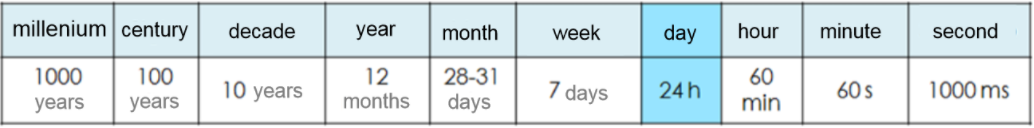As you can see in the table above, there are bigger and smaller units than a day. The smaller ones are hours, minutes and seconds; these are part of a sexagesimal system. This means that they go from 60 to 60, for example, an hour is 60 minutes and 1 minute is 60 seconds.

#### 1 h = 60 min     1 min = 60 s        1 hr = 3600 s

It’s also important to remember that these time measurements (sexagesimal system) can be expressed in two ways:

• Simple: using only one unit. For example: 8715 s
• Complex: using several units simultaneously. For example: 2 h 25 min 15 s. In the complex form, minutes and seconds can never surpass 60.

Is it possible to go from one type of unit to another?

The answer is yes. A measurement that’s written in the complex form can be written in the simplified form and vice versa. Let’s take a look at how to do that.

#### Time Measurement Problems: From Complex to Simple

We need to convert every part of the expression to the same unit (the one the problem asks for) and later, add them all together.

Express 2h 25 min 15 s in seconds: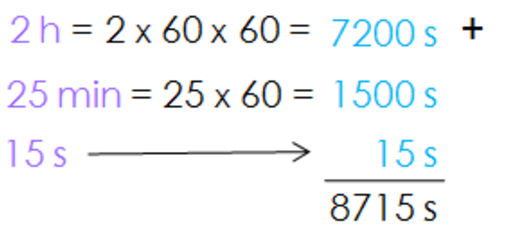#### Time Measurement Problems: From Simple to Complex

We need to divide the measurement and the successive quotient (if necessary) by 60.

Express 8715 s in the complex form: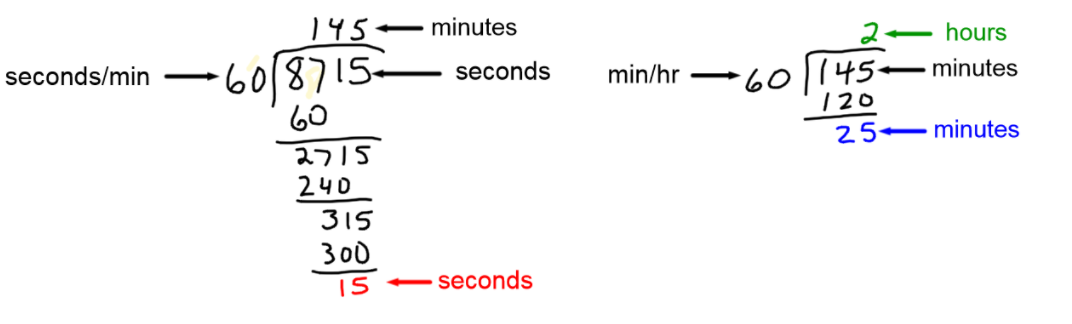#### 8715 s = 2h 25 min 15 s

In the example, we can see that the remainder of the first division is the seconds of the new expression, and the quotient (the minutes) is divided again by 60. The quotient of the second division is the hours and the minutes are the remainder.

With this information, we can now solve the following problem:

####The winner of the school’s go-kart race finished with a time of 1h 53 min 58 s. The racer in last place finished 365 s after the winner. What was the final time of the racer in last place? Express the answer in complex form.

We can see that the winner’s time is expressed in complex form and the difference of his time and the last qualifier’s time is expressed in simple form. The problem asks us to express the answer in the complex form, so the first thing we need to do is convert 365 seconds to the complex form.

In order to convert 365 seconds to the complex form, we have to start by dividing it by 60. The remainder that we get from dividing will be the seconds and the quotient, the minutes and because the number is smaller than 60, we don’t have to keep dividing.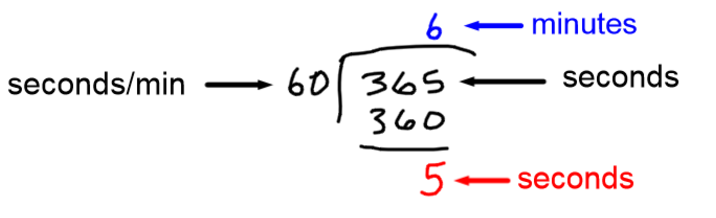#### 365 s = 6 min 5 s

The next step is to add everything in order to find out the total race time of the person that came in last place: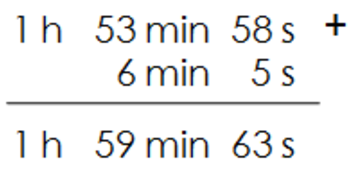Now, we add the seconds to the seconds, the minutes to the minutes, and the hours to the hours. The result is: 1h 59 min 63 s

So, are we done?

Not yet! Remember that in the complex form of time measurement, the seconds, and minutes must be less than 60. So, we still have to do one more step: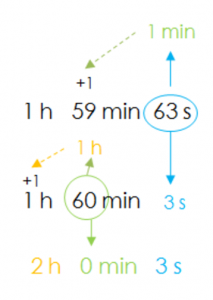63 s = 60 s + 3 s and 60 s = 1 min, therefore, we write 63 s as 1 min and 3 s.

We need to add that extra minute to the 59 minutes that we already had and we’re left with 1h 60 min 3 s.

Now we have 60 min and 60 min = 1 h

So then, 1 h 60 min 3 s = 2 h 0 min 3 s

And we finally have the answer expressed in the complex form: The total time of the last qualifier was 2 h 0 min 3 s

One last question before we finish…

####Is 1 min 30.132 s a correct expression?

Although it’s not very common, yes, it is a correct expression. In fact, it was the classification time of Fernando Alonso (Formula 1 racer) in Australia’s Grand Prix in 2014. He completed the track in 1 minute, 30 seconds, 1 hundredth, 3 tenths, and 2 milliseconds.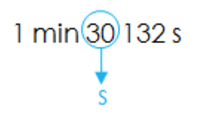The tenths, hundredths, and millionths of a second (submultiples of a second) are used when time needs to be measured extremely precisely, like in a Formula 1 race!

If you know of more situations that call for submultiples of a second, don’t think twice about letting us know and remember that you can log onto Smartick if you want to know more about time measurement problems.

By the way, one week is 7 days, or 168 hours, or 10080 minutes, or 604,800 seconds, or even 604, 800, 000 milliseconds!